# Class-XII Physics:Transient Current

## Growth and decay of current in LR circuit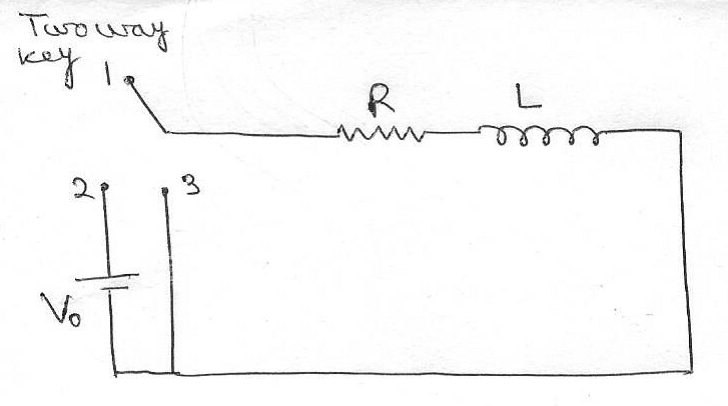## Growth of current

1 and 2 are connected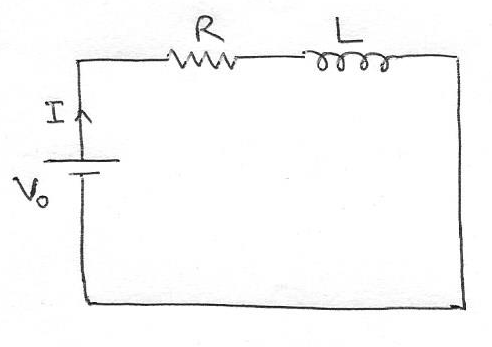• Current through circuit at any time t
1. At t =0, I = 0
2. At t = ∞ , I = Io = (Vo/R)
3. At any time t,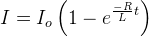where, τ = (L/R) = Inductive time constant
Io = maximum value of current in the circuit
At t = (L/R) ,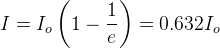• Voltage across Inductor (L) at any time t
1. At t =0, V = Vo
2. At t = ∞ , Vo = 0
3. At any time t,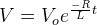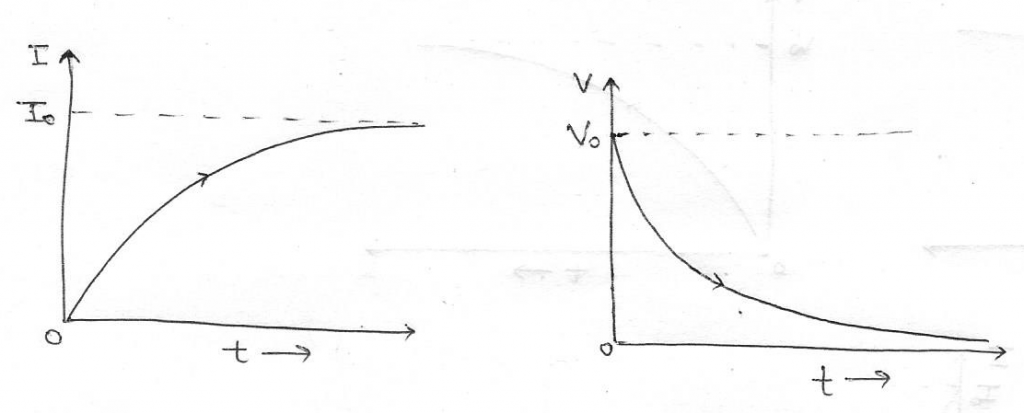• Energy stored in inductor at any time t
1. At t =0, U = 0
2. At t = ∞ ,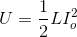= Uo
3. At any time t,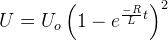• Heat produced in resistance in time t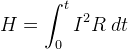## Decay of Current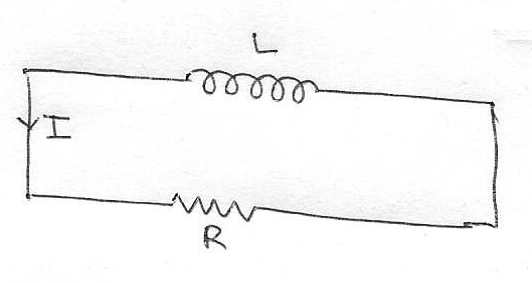1 and 3 are connected.
• Current through circuit at any time t
1. At t =0, I = Io = (Vo/R)
2. At t = ∞ , I = 0
3. At any time t,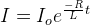where, τ = (L/R) = Inductive time constant
At t = (L/R) ,Inductive time constant of RL circuit is defined as the time during which the current in the circuit falls from maximum value to 0.368 times the maximum value of current.
• Voltage across Inductor (L) at any time t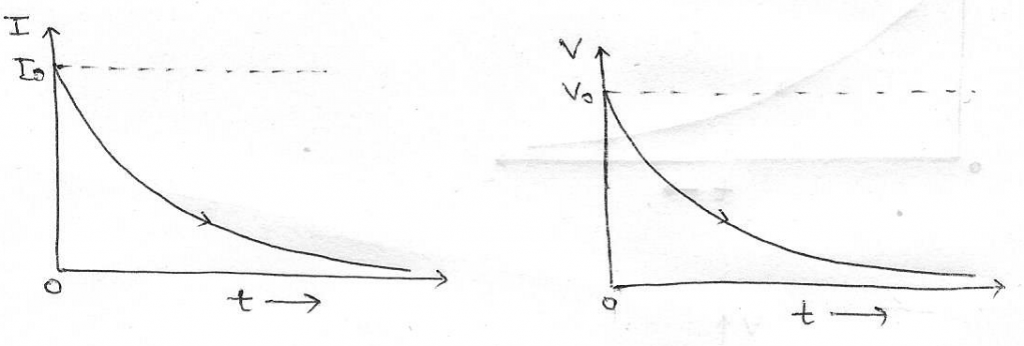• Energy remain in inductor (L) at any time t
1. At t =0,= Uo
2. At t = ∞ , U = 0
3. At any time t,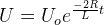• Heat produced in resistance (R) in time t= Uo – U

## Charging & discharging of a capacitor in RC circuit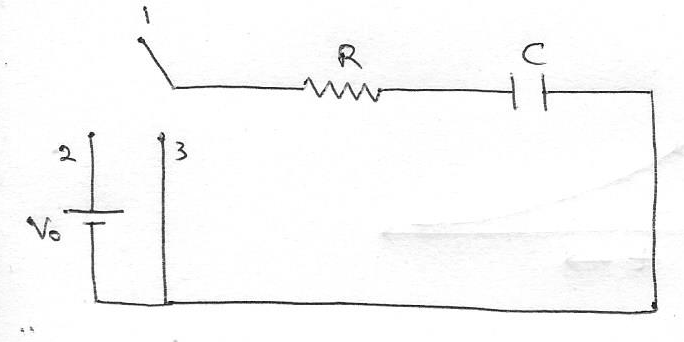## Charging of a capacitor in RC circuit

1 and 2 are connected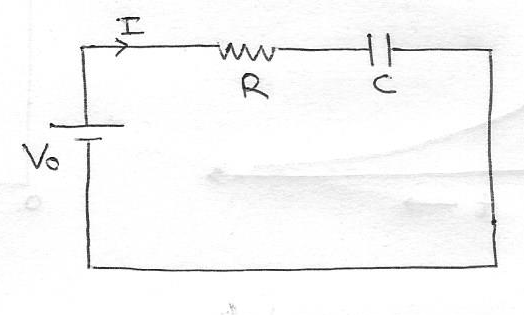• Charge at any instant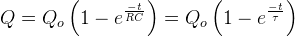τ = RC = Capacitive time constant
Qo = maximum value of charge
Capacitive time constant of RC circuit is defined as the time during which the charge in the capacitor grows to 0.632 times the maximum value of charge.
• Voltage across the capacitor during charging in RC circuit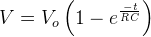• Current in RC circuit at any instant during the charging of the capacitor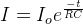Io = maximum value of current in the circuit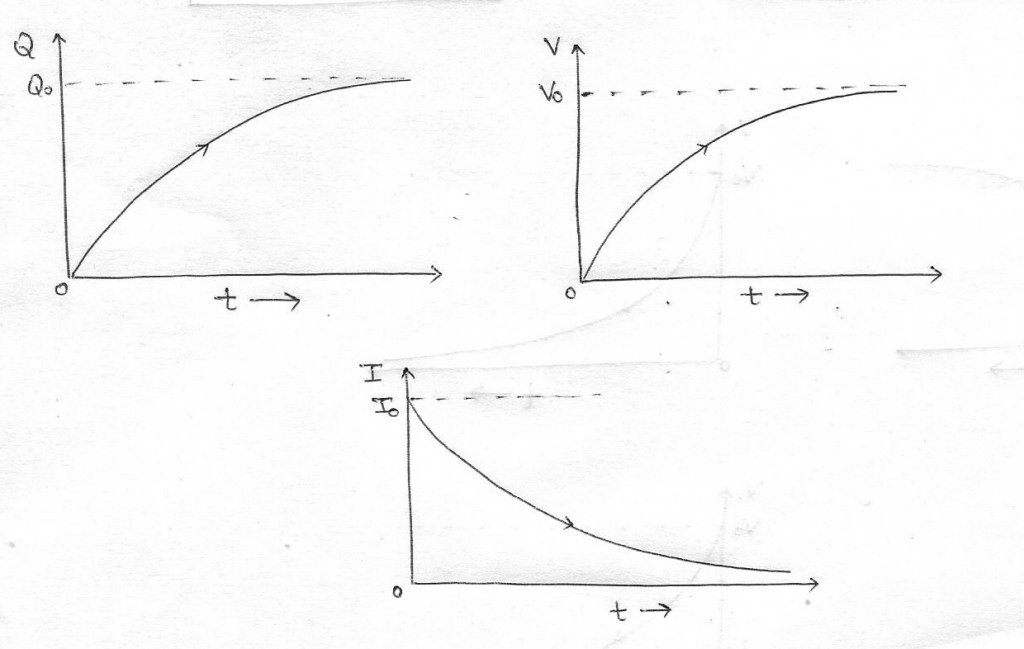## Discharging of a capacitor or decay of charge in RC circuit

1 and 3 are connected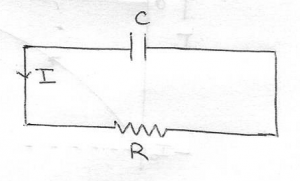• Charge at any instant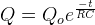Qo = maximum value of charge
• Voltage across the capacitor during discharging in RC circuit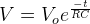• Current in RC circuit at any instant during the discharging of the capacitor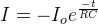Io = maximum value of current in the circuit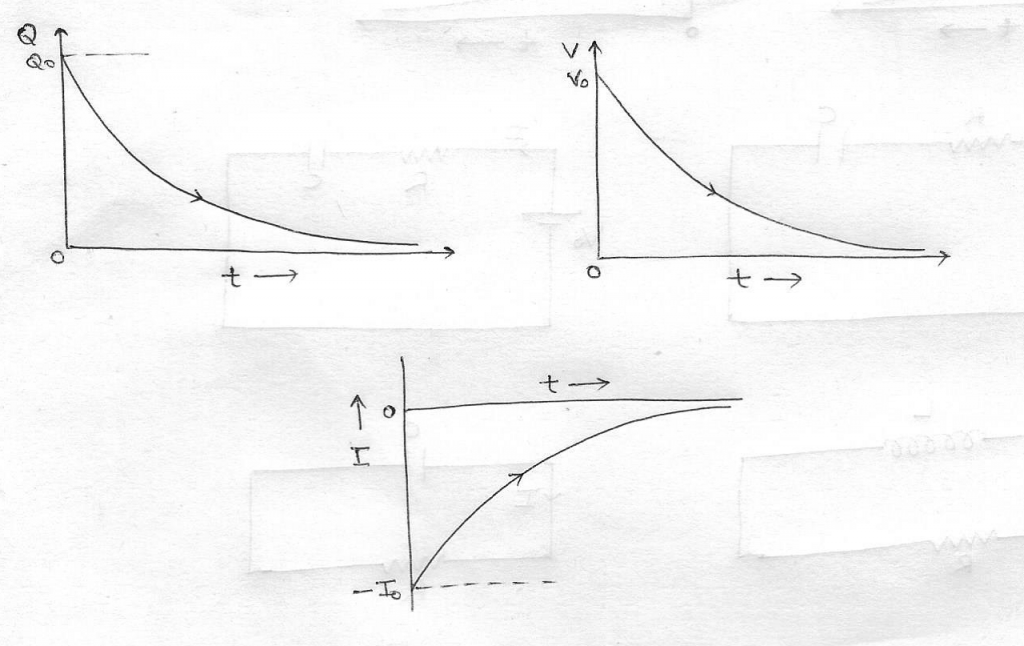This site uses Akismet to reduce spam. Learn how your comment data is processed.

error: Content is protected !!